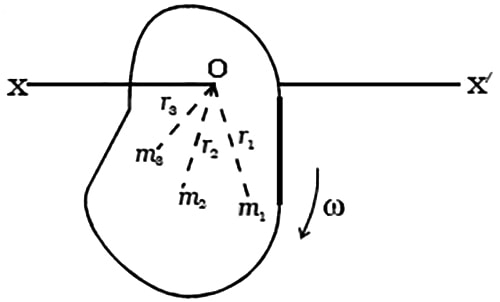# Explain Angular Momentum of a Rigid Body

Let consider a system of n particles of masses m1, m2 … …mn situated at distances r1, r2 … …r3 respectively from the axis of rotation (Figure).Let v1, v2, v3 … … be the linear velocities of the particles respectively, then the linear momentum of first particle = m1 v1.

Since v1 = r1 ω; the linear momentum of first particle = m1 (r1 ω)

The moment of linear momentum of first particle = linear momentum x perpendicular distance

= (m1 r1 ω) x r1

angular momentum of first particle = m1 r12 ω

Similarly,

Angular momentum of second particle = m2 r22 ω

Angular momentum of third particle = m3 r33 ω and so on.

The sum of the moment of the linear momentum of all the particles of a rotating rigid body taken together about the axis of rotation is known as the angular momentum of the rigid body.